## TRANSISTOR RF IMPEDANCE MATCHINGby Harry Lythall - SM0VPO## Introduction

In recent months I have been asked to provide more basic information, such as FET biasing, and before this; "how one can accurately calculate the impedance matching to power RF transistors?". In this article I will discuss transistor impedance maching but the article will not be entirely theoretical. There are a few points that are not so obvious from a purely mathematical point of view, for example bandwith.

As a matter of interest, when I took my basic RF engineers training it was all valves (tubes), where a high impedance of typically 5000 Ohms is matched to an output impedance of 50 Ohms. It was not until Cambridge 1975 to 1980 that I learned about transistors.

At first sight one knows that the input circuit to the output transistor has a pre-determined Q value, which in turn affects the RF drive to the transistors with frequency. BUT RF transistor specifications also give a specific power gain, which is subject to tolerance. In our example circuit I will assume the output transistor manufacturer has quoted a gain specification of 22dB. I don't believe that all transistors of one type can be relied upon to have the same gain, so I will overload the input circuit by 3dB to 5dB. This means that if the transistor does indeed fulfil its gain promise, then as the input circuit signal falls at band edges, the transistors will still be fed with 100% of the required signal instead of 300% (the 5dB extra drive).

The overall effect is that the output will remain flat(ish) and have a more predictable output power; independant if small transistors variations. The output matching circuit will, however, affect the output signal at band edges, but in commercial designs this is corrected by designing for a higher output power and using Automatic Gain Control (AGC) to keep the output flat.## Example circuit (VHF)

But enough chat, lets get on with some calculations. Here is my simple output stage for a 103MHz FM transmitter: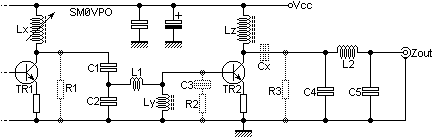Fig 1 - example exercise circuit.

Power Amplifier Data
Output power = 5 Watts
TR2 RF gain specification= 20dBm
Supply voltage = 13.8 Volts
Mode of bias = Class-C

Note that there are several components that are not numbered, for example Lx, Ly, and Lz, plus the odd DC-block or decoupling capacitor. These are not a part of the matching calculation so they will be covered seperately.

## Transistor output impedance

This is a rather difficult aspect to grasp for some people. One of the biggest sources of questions I get by e-mail is the 15-Watt HF linear power amplifier and the output coil matching. To match the collector of a device to an external load you need to know the load impedance and the transistor collector impedance from which it is to be fed. For any given output power the transistors delivers RF voltage and current. If the supply voltage is doubled then the current is halved and the collector impedance becomed four time higher: a square-law.

- For a single output transistor in class-A
the transistors collector impedance is the supply voltage squared divided by 4x the output power in watts.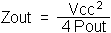- For a single output transistor in class-B or class-C
the transistors collector impedance is the supply voltage squared divided by 2x the output power in watts.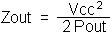- For a push-pull pair of transistors in class-A or Class-B
the output impedance is supply voltage squared divided by 2x the output power in watts.where:

• Vcc is the supply voltage to the stage
• Pout is the desired output power (Watts)
• Zout is the transistor collector impedance

In our circuit I have drawn R1 to represent the collector impedance of the driver transistor stage TR1. Practical RF power values for our amplifier would be 100mW from TR1 and 5W from TR2, which represents a 17dB gain in power for the output stage.

R1 = 12V x 12V / 2 x 0.1W = 144 / 0.2 = 720 Ohms (R1)
R3 = 12V x 12V / 2 x 5W = 144 / 10 = 14 Ohms (R3)

Note that I have used 12 Volts for Vcc and not 13.8 Volts. A nominal 12 Volt battery has a typicalterminal voltage between 12V and 14.5V. I have picked the lowest value.

## Matching TR1 collector to TR2 base

It is common practice to simply use two capacitors in series to form a high-to-low impedance converter. This I have done with C1 and C2. These are used to match the input of TR2 base. TR2 Base has a low input impedance, R2, but it also has a capacitive component, C3, that must be balanced out and this is done with L1. You can find the resistive and capacitive information in the transistor specifications. Take a closer look at the circuit: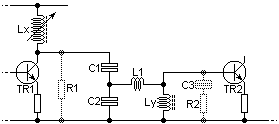Fig 2 - Matching TR1 to TR2.

I will use a Q value of 10 since I am only interested in a part of the FM band. So-far we have the values:

TR1 to TR2 values
R1 (TR1 output impedance) = 720 Ohms
R2 (TR2 input impedance)= 5 Ohms capacitive (from datasheet)
Q value = 10

The reactance of the inductor L1 is easy. Simply multiple R2 by the Q value you have chosen. In my example Xl = 10 x 5 = 50 Ohms.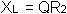Now use your basic inductance formula to find the value of inductance that will give you that impedance at your frequency of choice. In my example the inductor will be 0.07725969uH at 103MHz. Next we need to calculate C1 and C2.

The reactance of C1 is given by the following formula: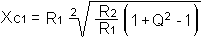The reactance of C2 is given by the formula: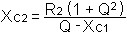The only component I have not discussed in the input circuit is the value of Lx. This should be chosen to have a reactance equal to R1 at the operating frequency. In this circuit Lx will resonate with C1 (in series with C2 to be precise) to form a band-pass tuned circuit so that C1 will not only become a part of the impedance matching network, but also a part of the RF alignment circuit. Lx should therefore be made variable by means of a ferrite tuning slug so that you can tune it. I usually make C1 variable but that is the cowards way out - too easy :)

## Matching TR2 collector to the output

The output impedance of the final stage is usually very low in transistor circuits, typically just a few Ohms. Once again the same formulas aply to determine TR2 output impedance:

- For a single output transistor in class-A
the transistors collector impedance is the supply voltage squared divided by 4x the output power in watts.- For a single output transistor in class-B or class-C
the transistors collector impedance is the supply voltage squared divided by 2x the output power in watts.- For a push-pull pair of transistors in class-A or Class-B
the output impedance is supply voltage squared divided by 2x the output power in watts.where:

• Vcc is the supply voltage to the stage
• Pout is the desired output power (Watts)
• Zout is the transistor collector impedance

So now we know the output impedance of TR2 we can look at the component values. Consider now this part of the circuit: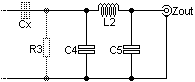Fig 3 - Matching TR2 to output.

In our circuit I have drawn R3 to represent the collector impedance of the output transistor stage TR2. The output circuit from the final stage is a simple PI filter comprising C4, L2 and C5. The value of C4 is given by the formula:

The value of C4 is given by the formula: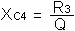Remember also that this capacitor includes TR2 output capacitance. If for example TR2 had a 10pf output capacitance and you needed 40pf for C4, then you would fit 40pf - 10pf = 30pf component in your circuit.

The value of L2 is given by the formula: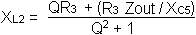The value of C5 is given by the formula: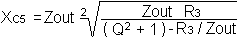## The unwritten components

If we now go back to the original circuit you will see that there are several components we have not yet covered: Lx, Ly, Lz and Cx. There is also an unmarked resistor in the emitter of each RF transistor.Fig 1 - example exercise circuit.

Lx is often tuned and the capacitance of the output transistor + C1 make up the tuned circuit. If you want to be extremely exact then Lx resonates with (C1 x C2) / (C1 + C2) but since C2 is usually so much larger than C1 the value can be assumed to be C1. To be accurate then the inductor should be tuned but in practice you can make C1 variable. You may have to make a minor change to Lx.

Ly has an impedance that is 10x R2 or more. The bigger the better. This is normally about 2 to 5 turns on a small ferrite bead. Its job is to bias the output transistor to 0v (ground).

Lz is normally 10x the impedance of TR2 collector (R3) and is just an RF choke. This is often about 10 to 20 turns of thick wire enamelled wire about 8mm Dia. Ferrite is not normally used here because of the high powers. If you are designing for powers of less than about 1 Watt then you can use ferrite beads, which will improve stability.

Cx is normally chosen to be about 5% of the impedance of TR2 collector (R3) as A MINIMUM. This capacitor may typically have a value of 1nf 10nf, 100nf, or anywhere between. The bigger the better, as long as the capacitor's self inductance does not become relevant to the other circuit values.

The unmarked resistor in the emitter of each transistor is normally 10 or 22 ohms for low power devices and typically less than 1 Ohm for modest power devices. It has several functions: it increases linearity and helps to prevent the transistor from being overloaded. It also "evens out" the gain of transistors so that, for example, push-pull devices working in tandem give equal shares of the output load. The resistor is normally calculated to give about 1-volt drop with full current load. It also increases the negative feedback a little so that the gain is slightly reduced but more stable.A lot easier than it sounds. All you have to do is work out the transistor output impedance and the impedance you want to load. Then wind a broadband transformer on ferrite that will have that impedance ratio (square root of impedance ratio = turns ratio).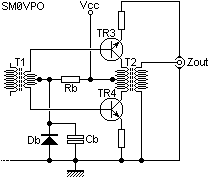As usual, the problem is to get a decent calculation for the transistor output impedance, but it is the same as those above:

- For a single output transistor in class-A
the transistors collector impedance is the supply voltage squared divided by 4x the output power in watts.- For a single output transistor in class-B or class-C
the transistors collector impedance is the supply voltage squared divided by 2x the output power in watts.- For a push-pull pair of transistors in class-A or Class-B
the output impedance is supply voltage squared divided by 2x the output power in watts.where:

• Vcc is the supply voltage to the stage
• Pout is the desired output power (Watts)
• Zout is the transistor collector impedance

For broadband amplifiers a LOT depends on the frequency and purely mechanical considerations. For example. The inductive impedance of the transistors collector winding must be about 5x the transistor output impedance at the lowest operating frequency.

At the upper operating frequency it is your construction technique that limits the response. The inter-turn capacitance of the secondary winding (or the highest impedance winding) is usually that which limits the upper frequency. Surprisingly, enamelled magnet wire has a higher inter-winding capacitance than PTE or PTFE covered wire. I found this out in practice. I presume that the plastic forces a larger gap between conductors.

Typical values for T1 are 10 turns for the primary and 1/2 + 1/2 turn for the secondary. T1 would normally be wound on a ferrite bead having two parallel holes. One turn is when a wire is passed through one hole and back through the other hole. This will match anything like 100 Ohms to the base of TR1 and TR2.

The unmarked resisistors in the emitter of TR1 and TR2 are designed to drop about vDC with maximum collector current. They are there for stability and to ballance the two output transistors.

Rb, Db and Cb are nothing more than bias components for the output transistors. This may be simple, as shown, but for higher power amplifiers it may consist of a really heavy series regulator with thermal compensation.## References

If you want more information then these publications may help you a lot. Some I believe to be over-complicated but if you want to learn more then AN721 is both informative and readable. My PYE-Telecom training in 1977 gave exactly the same formulas :-). but the matching networks I was taught were a little more simplified.

1. Motorola Semiconductors AN721
2. PYE Telecommunications (Cambs UK), training dept course handouts (1977)
3. Secrets of RF Circuit Design by Joseph J Carr.
4. 2N3866 Datasheet by NXP Semiconductors (Philips), Kista, Sweden.